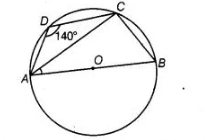# ABCD is a cyclic quadrilateral`
Question:

ABCD is a cyclic quadrilateral such that AB is a diameter of the circle circumscribing it and ∠ADC = 140°, then ∠BAC is equal to

(a) 80°

(b) 50°

(c) 40°

(d) 30°

Solution:

We know that, sum of the opposite angles in a cyclic quadrilateral is 180°.We know that, sum of the opposite angles in a cyclic quadrilateral is $180^{\circ}$.

$\angle A D C+\angle A B C=180^{\circ}$

$\Rightarrow \quad 140^{\circ}+\angle A B C=180^{\circ}$

$\Rightarrow \quad \angle A B C=180^{\circ}-140^{\circ}$

$\therefore \quad \angle A B C=40^{\circ}$

Since, $\angle A C B$ is an angle in a semi-circle.

$\therefore \quad \angle A C B=90^{\circ}$

In $\triangle A B C, \quad \angle B A C+\angle A C B+\angle A B C=180^{\circ}$

[by angle sum property of a triangle]

$\Rightarrow \quad \angle B A C+90^{\circ}+40^{\circ}=180^{\circ}$

$\Rightarrow \quad \angle B A C=180^{\circ}-130^{\circ}=50^{\circ}$